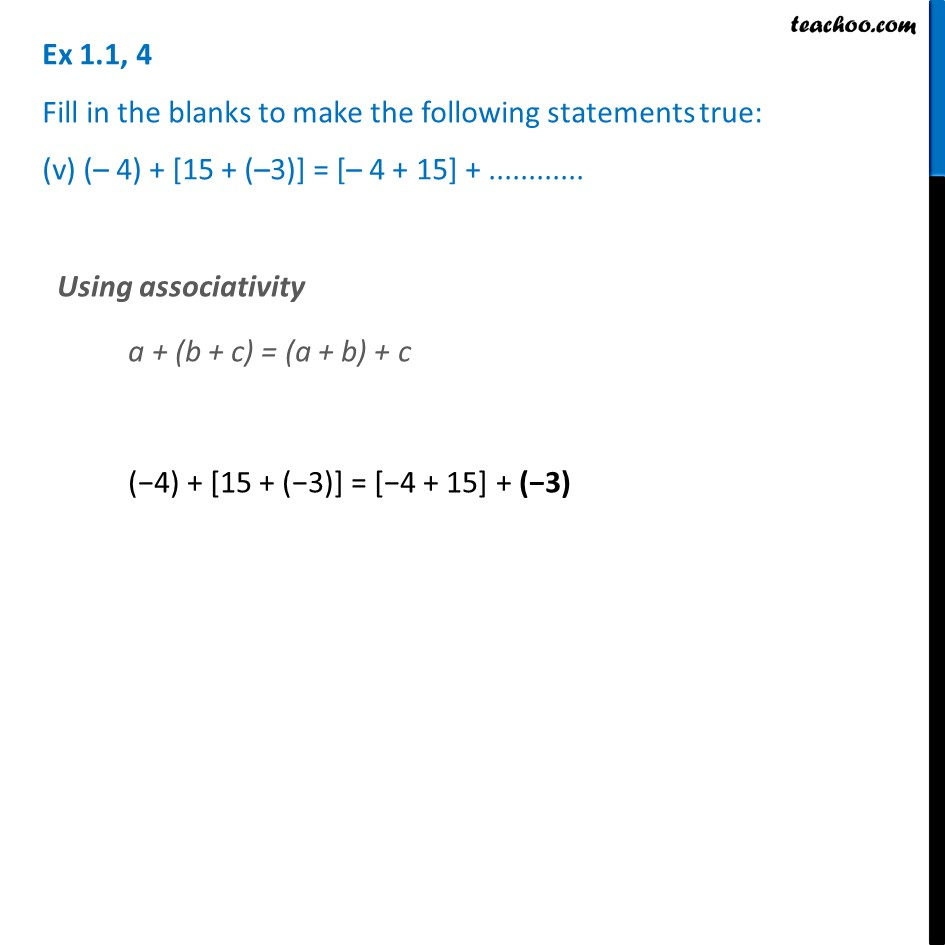Ex 1.1

Chapter 1 Class 7 Integers
Serial order wiseLearn in your speed, with individual attention - Teachoo Maths 1-on-1 Class

### Transcript

Ex 1.1, 4 Fill in the blanks to make the following statements true: (v) (– 4) + [15 + (–3)] = [– 4 + 15] + ............ Using associativity a + (b + c) = (a + b) + c (−4) + [15 + (−3)] = [−4 + 15] + (−3)